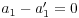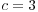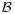# Coordinates and Change of Basis

Let V be a vector space and letbe a basis for V. Every vectorcan be uniquely expressed as a linear combination of elements of:(Let me remind you of why this is true. Since a basis spans, everycan be written in this way. On the other hand, ifare two ways of writing a given vector, then, and by independence, ...,--- that is,, ...,. So the representation of a vector in this way is unique.)

Consider the situation whereis a finite ordered basis --- that is, fix a numberingof the elements of. If, the ordered list of coefficientsis uniquely associated with v. Theare the components of v with respect to the (ordered) basis; I will use the notationIt is easy to confuse a vector with the representation of the vector in terms of its components relative to a basis. This confusion arises because representation of vectors which is most familiar is that of a vector as an ordinary n-tuple in:This amounts to identifying the elements ofwith their representation relative to the standard basisExample. (a) Show thatis a basis for.

These are three vectors in, which has dimension 3. Hence, it suffices to check that they're independent. Form the matrix with the elements ofas its rows and row reduce:The vectors are independent. Three independent vectors inmust form a basis.(b) Find the components ofrelative to.

I must find numbers a, b, and c such thatThis is equivalent to the matrix equationSet up the matrix for the system and row reduce to solve:This says,, and. Therefore,.(c) Writein terms of the standard basis.

I'll writefor v relative toandfor v relative to the standard basis. The matrix equation in (b)saysIn (b), I knewand I wanted; this time it's the other way around. So I simply putinto thespot and multiply:Let me generalize the observation I made in (c).

• Ifis a basis for--- whose elements are written in terms of the standard basis, of course --- and M is the matrix whose columns are the vectors in, then left multiplication by M translates vectors written in terms ofto vectors written in terms of the standard basis.I'll writefor M, and call it a translation matrix. Again,translates vectors written in terms ofto vectors written in terms of the standard basis.

The inverse of a square matrix M is a matrixsuch that, where I is the identity matrix. If I multiply the last equation on the left by, I getIn words, this means:

• Left multiplication bytranslates vectors from the standard basis to.

This means that. Dispensing with M, I can say thatIn the example above, left multiplication by the following matrix translates vectors fromto the standard basis:The inverse of isLeft multiplication by this matrix translates vectors from the standard basis to.

Example. (Translating vectors from one basis to another) The translation analogy is a useful one, since it makes it easy to see how to set up arbitrary changes of basis.

For example, supposeis another basis for.

Here's how to translate vectors fromto:Remember that the productis read from right to left! Thus, the composite operationtranslates avector to a standard vector, and then translates the resulting standard vector to avector. Moreover, I have matrices which perform each of the right-hand operations.

This matrix translates vectors fromto the standard basis:This matrix translates vectors from the standard basis to:Therefore, multiplication by the following matrix will translate vectors fromto:Contact information

Copyright 2008 by Bruce Ikenaga